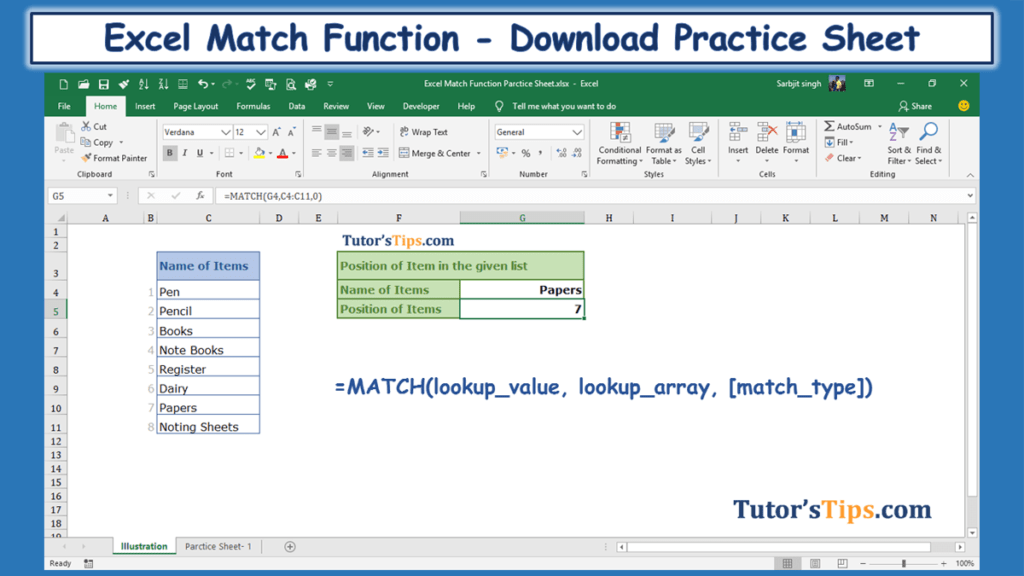## Excel Match Function:-

The Excel Match function is used to find out the position of the selected value in the selected array. The position of the selected value may be exact matched, less than and more than from the value.

### The feature of “Match” function: –

• With the help of Match Function, we can retrieve the position of the Selected value in the selected array and we can use this position further with index function and others also.
• It helps in getting a particular position of a certain value in the selected array.

### Explanation of Function:-

Now, We will explain the Arguments of the Function.

`=MATCH(lookup_value, lookup_array, [match_type])`

lookup_value: –

The lookup_value that value of which we want to know the position in the selected array.

lookup_array: –

The lookup_array that selected cells from which we want to know the position of the lookup_value.

match_type: –

The match_type are of three types show as following: –

1.  If we put the value “1”  – The MATCH function will find the largest value that is less than or equal to value. You should be sure to sort your array in ascending order.
2. If we put the value “0”  –  The MATCH function will find the first value that is equal to value. The array can be sorted in any order.
3. If we put the value “-1” – The MATCH function will find the smallest value that is greater than or equal to value. You should be sure to sort your array in descending order.

### Example of Function: –

I will show you, how to apply the whole function. (using optional also).

From the following table get the position of the papers in the selected array: –

Solution:-

We will Apply the Match function and get the result. This is shown in the following steps: –

Step No. 1:-

We will write the “=Match( ” in the column of the result.

Step No. 2

Now select the cells with which you want to match the value with the selected array.

Step No. 3

Now select the array.

Step No. 4

Now apply matching condition

Step No. 5: –

Now, Copy and paste the function in all remaining cell to get the result.